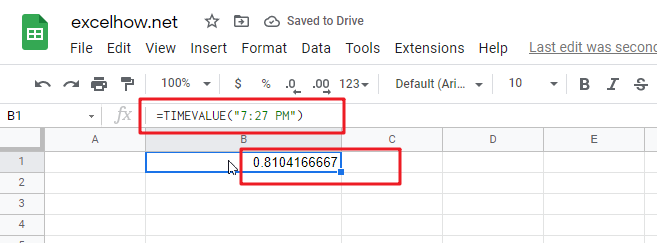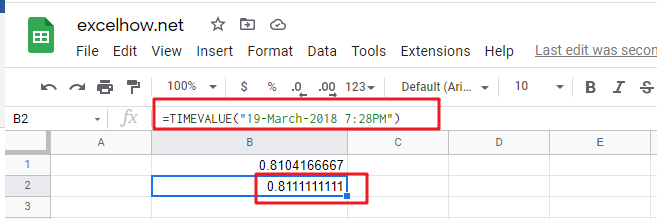# ExcelHow

This post will guide you how to use Google Sheets TIMEVALUE function with syntax and examples.

## Description

The Google Sheets TIMEVALUE returns the decimal number of the time represented by a text string. It will convert a time represented by a text string into a time value. and the returned value is range from 0 to 0.99988, it indicated the times from 0:00:00 to 23:59:59.

For example, when you fed the text string “7:13 PM” into TIMEVALUE function, and it returns 0.80069. the returned value is a numeric value and this value can be used to manipulate with another formulas.

The TIMEVALUE function can be used to get the fraction of a 24-hour day the time represents. The purpose of this function is to get a valid time from a text string and its returned values is a valid time as a decimal number.

The TIMEVALUE function is a build-in function in Google Sheets and it is categorized as a Date function.

## Syntax

The syntax of the TIMEVALUE function is as below:

=TIMEVALUE (time_text)

Where the TIMEVALUE function arguments is:

• time_text -This is a required argument. A text string that represents a time, within the text string, the hours, minutes and seconds components should be separated by colon characters.

Note:

• the date information in text string will be ignored.
• if the time_text is not a valid time in a text format , it will return #VALUE! error.

## Google Sheets TIMEVALUE Function Examples

The below examples will show you how to use Google sheets TIMEVALUE Function to convert a text string that representing a time in Excel.

1# get decimal part of a day, with only the time portion specified, enter the following formula in Cell B1.

`=TIMEVALUE("7:27 PM")`2# get decimal part of a day, with date and time specified, enter the following formula in Cell B3.

`=TIMEVALUE("19-March-2018 7:28PM")`